# Programming with VBA n n n Planning for

• Slides: 22
Download presentationProgramming with VBA n n n Planning for Programming Variables and Constants Assignment Statements Functions Programming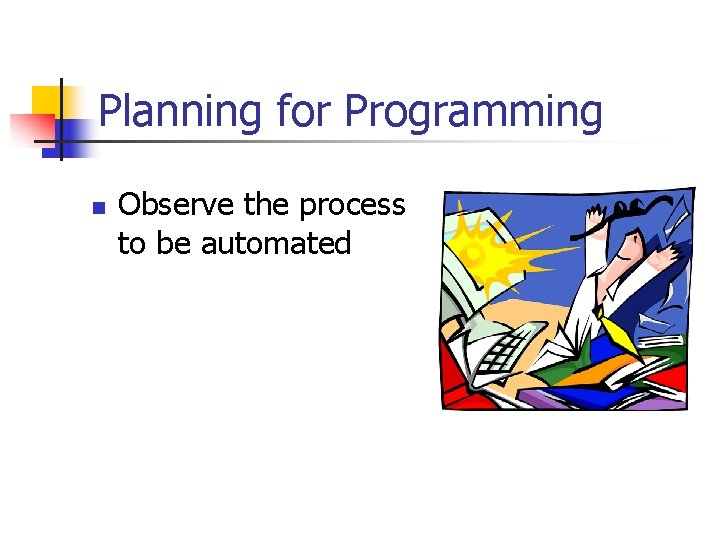Planning for Programming n Observe the process to be automated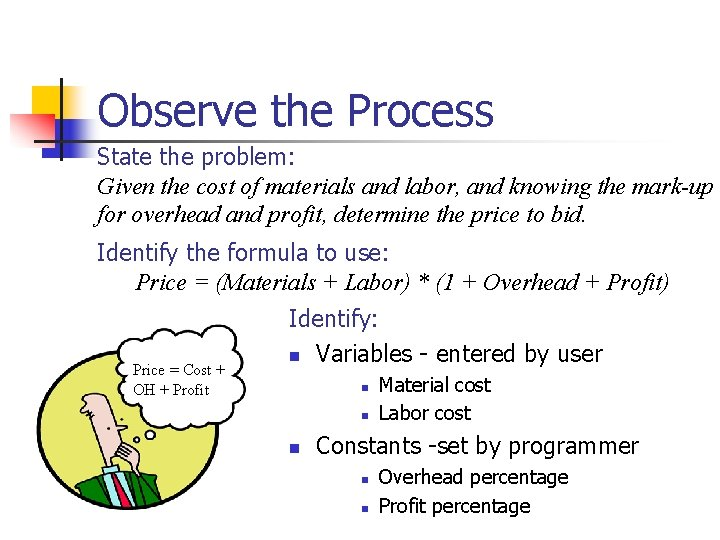Observe the Process State the problem: Given the cost of materials and labor, and knowing the mark-up for overhead and profit, determine the price to bid. Identify the formula to use: Price = (Materials + Labor) * (1 + Overhead + Profit) Price = Cost + OH + Profit Identify: n Variables - entered by user n n n Material cost Labor cost Constants -set by programmer n n Overhead percentage Profit percentage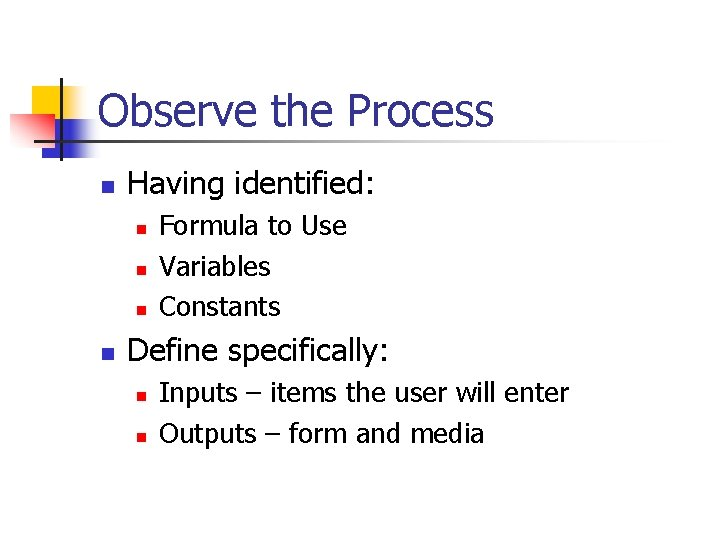Observe the Process n Having identified: n n Formula to Use Variables Constants Define specifically: n n Inputs – items the user will enter Outputs – form and media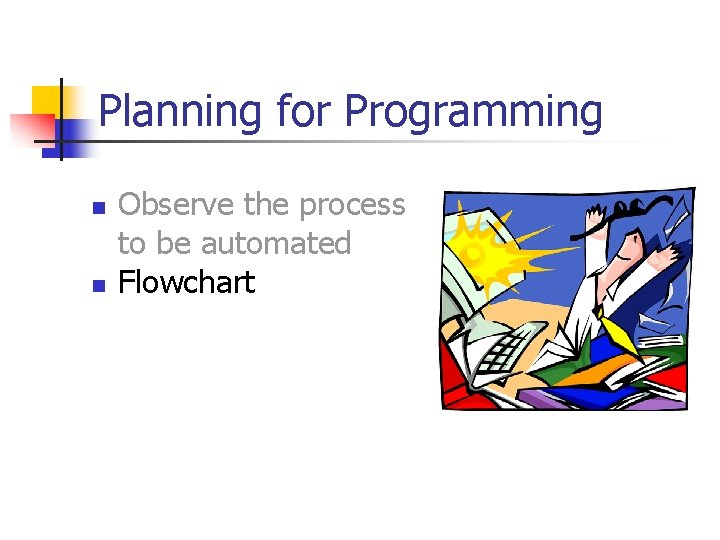Planning for Programming n n Observe the process to be automated FlowchartFlow Charts n n Schematic Drawing of Process Use Standard Symbols Flow from Top to Bottom Connect with Arrows Start or Stop Data Input/Output Process steps Predefined Process Decisions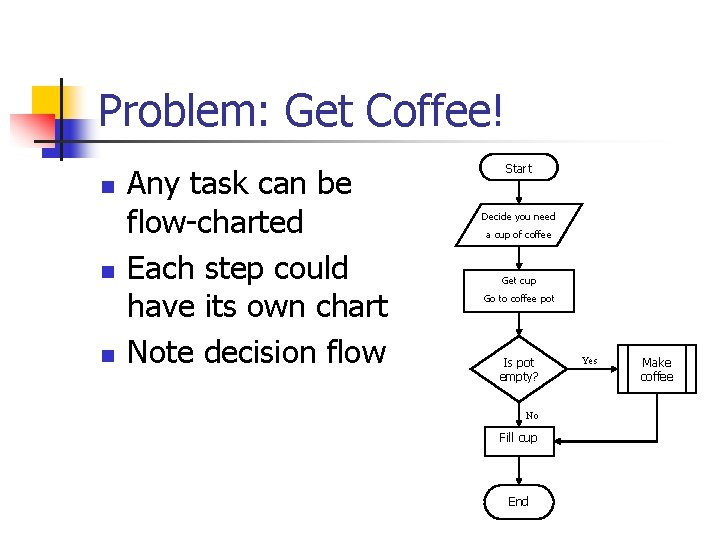Problem: Get Coffee! n n n Any task can be flow-charted Each step could have its own chart Note decision flow Start Decide you need a cup of coffee Get cup Go to coffee pot Is pot empty? No Fill cup End Yes Make coffeeCost Model n n Start Get user inputs n n n Material Labor Determine cost Determine price Output results Quit Start Get: Labor cost Material cost Determine: Total cost Bid Price Show Bid Price QuitPlanning for Programming n n Observe the process to be automated Flowchart Write Pseudo Code Edit Code with VBA Editor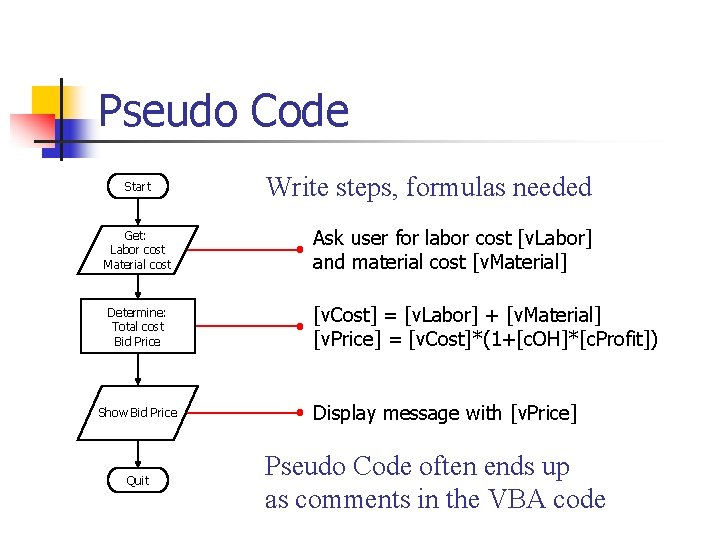Pseudo Code Start Get: Labor cost Material cost Determine: Total cost Bid Price Show Bid Price Quit Write steps, formulas needed Ask user for labor cost [v. Labor] and material cost [v. Material] [v. Cost] = [v. Labor] + [v. Material] [v. Price] = [v. Cost]*(1+[c. OH]*[c. Profit]) Display message with [v. Price] Pseudo Code often ends up as comments in the VBA code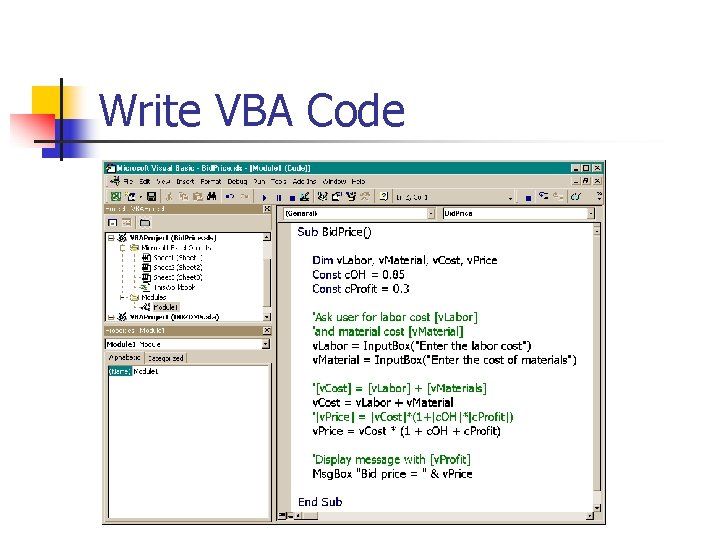Write VBA CodeVariables and Constants n n n Storage “bins” in memory Identified by a unique name Variables can be changed by the user n n v. Name = Input. Box(“Enter your name”) Constants can only be changed by editing the VBA code n Const c. Mark. Up = 0. 25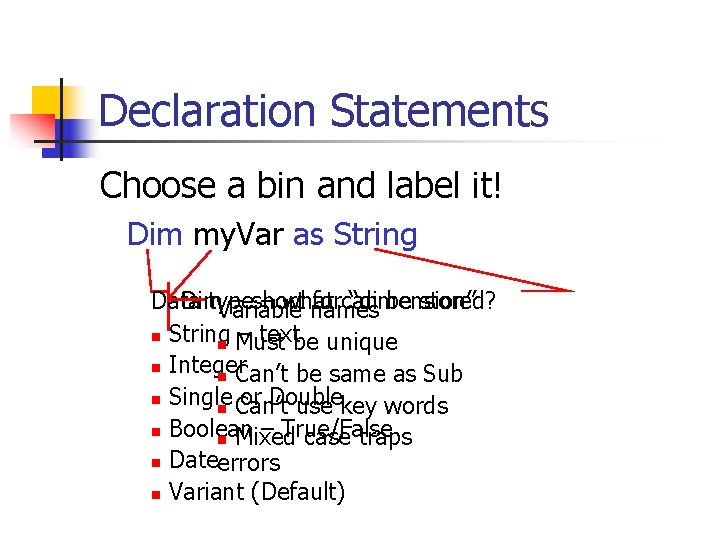Declaration Statements Choose a bin and label it! Dim my. Var as String Data Dim type – short – what forcan “dimension” be stored? Variable names n String – text n Must be unique n Integer n Can’t be same as Sub n Single or Double n Can’t use key words n Boolean – True/False n Mixed case traps n Dateerrors n Variant (Default)Declaration and Scope n Procedure Level – Dim n n Module Level n n n Dim v. Name as Text Place below Sub() Value resets to Empty after End Sub Place ahead of all Sub() statements Value from one Sub() available in others All procedures in all Modules – Public n Public v. Profit as SingleConstants n Declarations n n n Const my. Dogs as Integer = 4 Must appear before assignment statements Built-in n Typically named “vb? ? ” n n n vb. Yes vb. Red Look at Help under Constants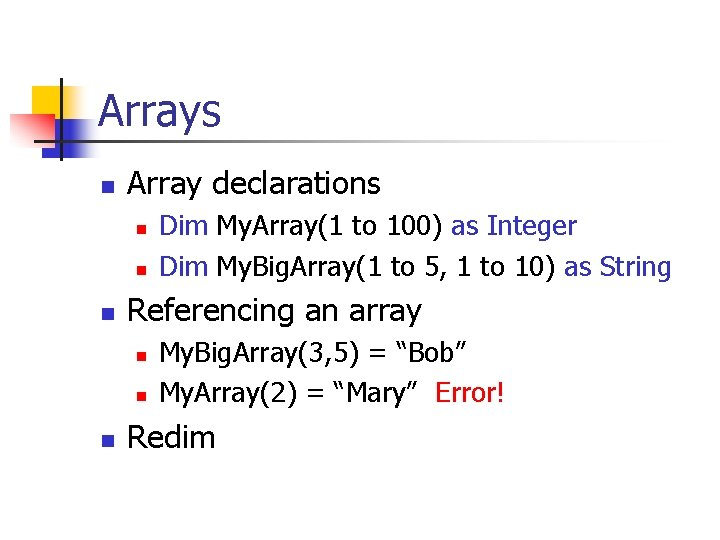Arrays n Array declarations n n n Referencing an array n n n Dim My. Array(1 to 100) as Integer Dim My. Big. Array(1 to 5, 1 to 10) as String My. Big. Array(3, 5) = “Bob” My. Array(2) = “Mary” Error! Redim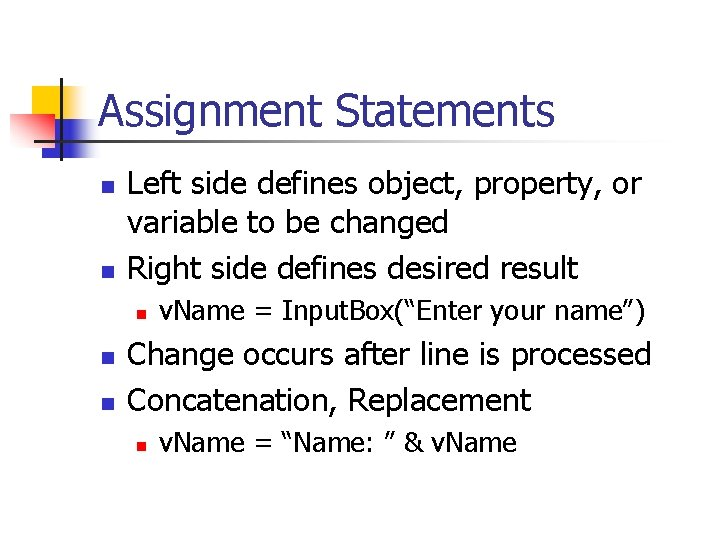Assignment Statements n n Left side defines object, property, or variable to be changed Right side defines desired result n n n v. Name = Input. Box(“Enter your name”) Change occurs after line is processed Concatenation, Replacement n v. Name = “Name: ” & v. Name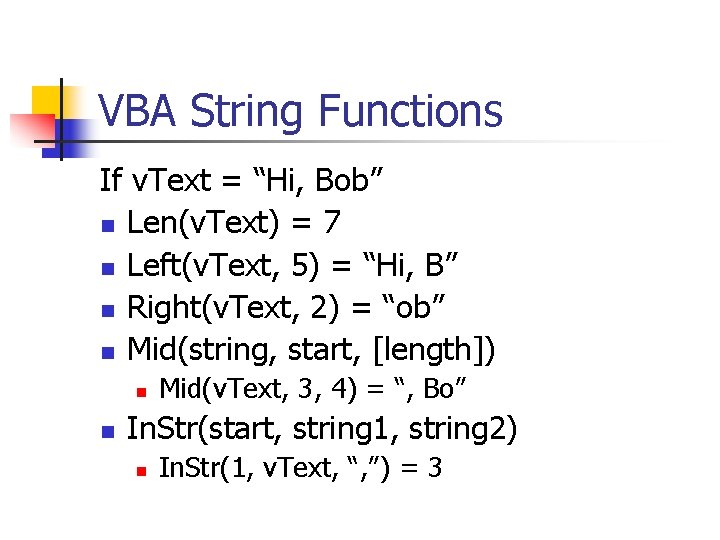VBA String Functions If v. Text = “Hi, Bob” n Len(v. Text) = 7 n Left(v. Text, 5) = “Hi, B” n Right(v. Text, 2) = “ob” n Mid(string, start, [length]) n n Mid(v. Text, 3, 4) = “, Bo” In. Str(start, string 1, string 2) n In. Str(1, v. Text, “, ”) = 3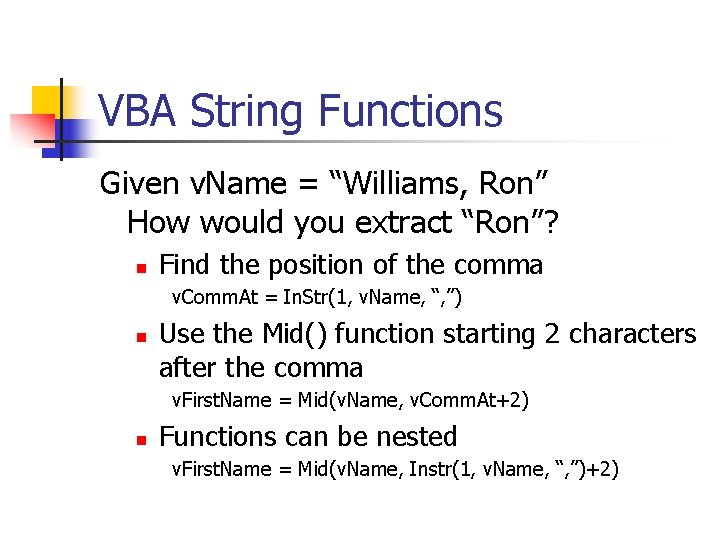VBA String Functions Given v. Name = “Williams, Ron” How would you extract “Ron”? n Find the position of the comma v. Comm. At = In. Str(1, v. Name, “, ”) n Use the Mid() function starting 2 characters after the comma v. First. Name = Mid(v. Name, v. Comm. At+2) n Functions can be nested v. First. Name = Mid(v. Name, Instr(1, v. Name, “, ”)+2)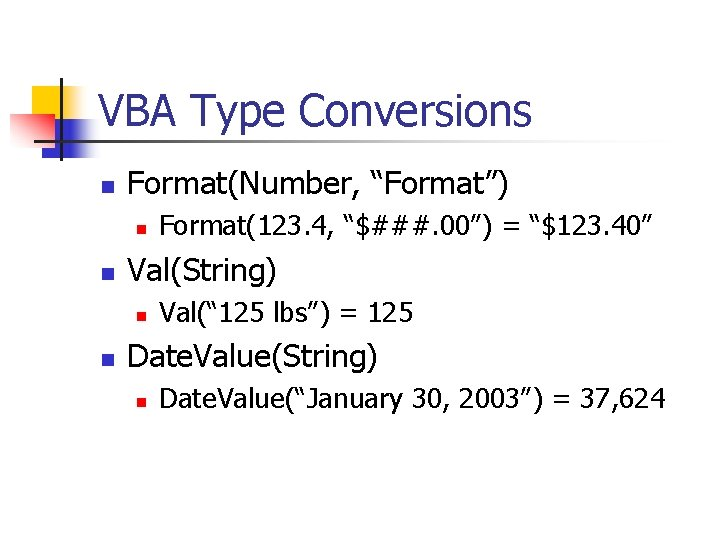VBA Type Conversions n Format(Number, “Format”) n n Val(String) n n Format(123. 4, “\$###. 00”) = “\$123. 40” Val(“ 125 lbs”) = 125 Date. Value(String) n Date. Value(“January 30, 2003”) = 37, 624Methods n Actions to be taken n n n Select Copy Paste Clear Delete Connect to object with period n Range(“B 3”). Clear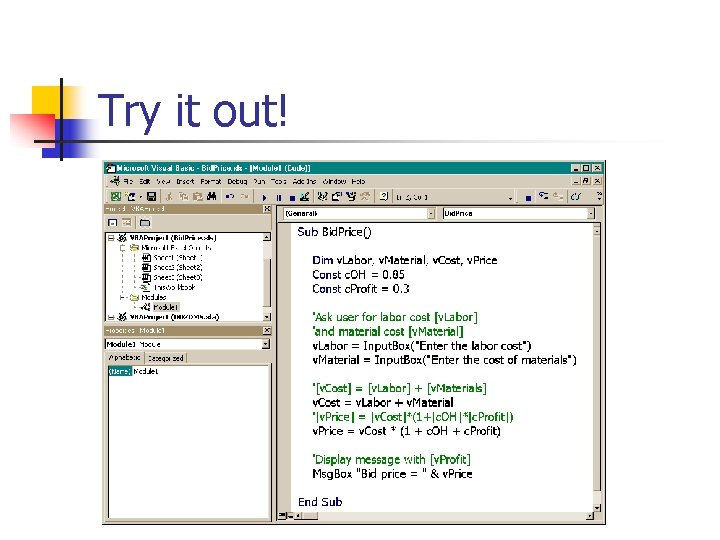Try it out!